output.to from Sideway
Mechanics: Statics

Draft for Information Only

# Content

``` Internal Forces  Internal Forces in Members   Straight Two-force Member    Multi-force Member   Two-force Frame Member```

# Internal Forces

When an rigid body is in static equilibrium, the external forces acting on the rigid body is in equilibrium also. And the members of a static equilibrium structure is in static equilibrium also. When a member is in static equilibrium, the internal forces in the member tending to resist those external forces should be in equilibrium also.

The external forces exert either tension or compression forces on the straight two-force truss member of a truss. There is no moment acting on the member because the line of force acts along the axis of the structure member. The only possible internal forces to react and hold the memeber structure in equilibrium is tension or compression forces also. But when the external forces exert either tension or compression forces on the multiforce truss member of a frame or machine structure, there may have moment acting on the member because the line of force does not alway act along the axis of the structure member or the axis of the sturcture member is not a straight line. These external force usually produce shear forces and bending moments on the structure member and therefore the internal forces to react and hold the member structure in equilbrium is tension or compression forces and together with shear and bending as well.

## Internal Forces in Members

### Straight Two-force Member

For a straight two-force member, the two external forces at the two end of a truss member should be equal in magnitude and line of action but opposite in sense when the truss member is in equilibrium. The internal forces in truss member can be determined by assuming the member is being cut by an imaginary section plane.

For example a straight two-force member in tensionSince the truss member is in equilibrium, tension force F at point A and tension force F' at point B should be equal in magnitude but opposite in sense. For a truss member in equilibrium, the internal forces at the imaginary section plane C should also be in equilibrium. Therefore, the truss member AB can be considered as two separated equilibrium free body models, member section AC and member section CB. Imply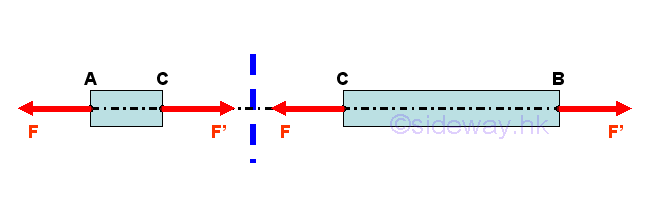Since the two seperated equilibrium free body models, member section AC and member section CB are in equilibrium, the internal forces reacts by the imaginary section plane C on member section AC and member section CB must equal to external forces on each member. Therefore a force F' which equivalent to the internal force and is equal and opposite to force F should be applied to member section AC at C. And a force F which equivalent to the internal force and is equal and opposite to force F' should be applied to member section CB at C. The internal forces on the two seperated member sections AC and CB should alway exert on each other in truss member AB and are equal in magnitude but opposite in sense. For the straight two-force member, the internal forces are only axial forces along the axis of the straight two-force member, the location of the imaginary section plane C does not affect the magnitude of the internal forces in member AB.

When the straight two-force member in compressionThe internal forces can be determined in similar way.### Multi-force Member

For a multi-force member, the external forces acting on the frame member should be equilibrium when the frame member is in equilibrium. The internal forces in frame member can be determined by assuming the member is being cut by an imaginary section plane. For example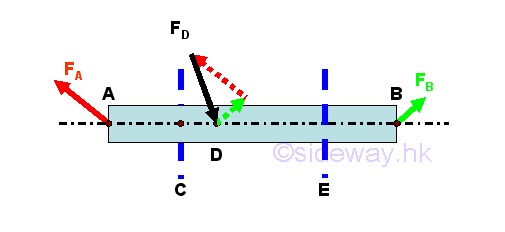Forces are in equilibrium, imply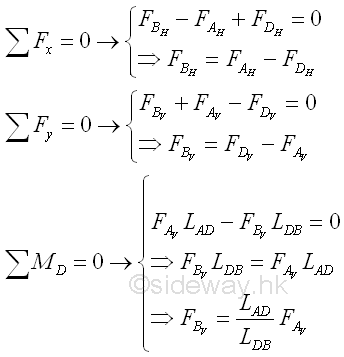Since the frame member is in equilibrium, The sum of external forces applied to point B and point C should be equal in magnitude but opposite in sense to the external force F at point A. For a frame member in equilibrium, the internal forces at the imaginary section plane C should also be in equilibrium. Therefore, the frame member AB can be considered as two separated equilibrium free body models, member section AC and member section CB. ImplySince the two seperated equilibrium free bodies, member section AC and member section CB are in equilibrium, the internal forces reacts by the imaginary section plane C on member section AC and member section CB must equal to external forces on each member. Therefore a system of forces which equivalent to the internal force and is equal and opposite to force FA should be applied to member section AC at C. And a system of forces which equivalent to the internal force and is equal and opposite to the sum of force FB and FD should be applied to member section CB at C. The internal forces on the two seperated member sections AC and CB should alway exert on each other in truss member AB and are equal in magnitude but opposite in sense. For the straight multi-force member, the internal forces are a system of forces and moment which can be transformed to a force-couple system along the axis of the straight multi-force member, the location of the imaginary section plane C will affect the magnitude of the force-couple system of the internal forces in member AB because the resultant force of the internal forces is affected by the location of application of the external forces and the resultant moment of the internal forces is also affected by the location of interest at which moment of the external forces acting on.

Consider the member section AC of length LAC, the internal forces at point C areConsider another member section AE of length LAE,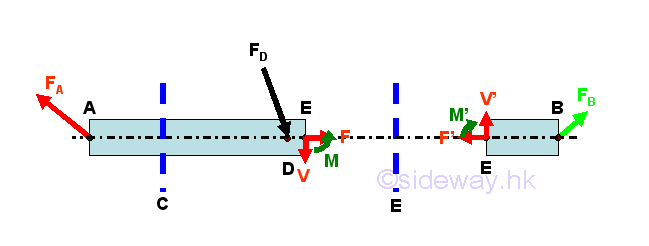Since the internal forces at the imaginary section plane E should be in equilibrium, the internal forces at point E can also be determined using the member section EB of length LEB,  Imply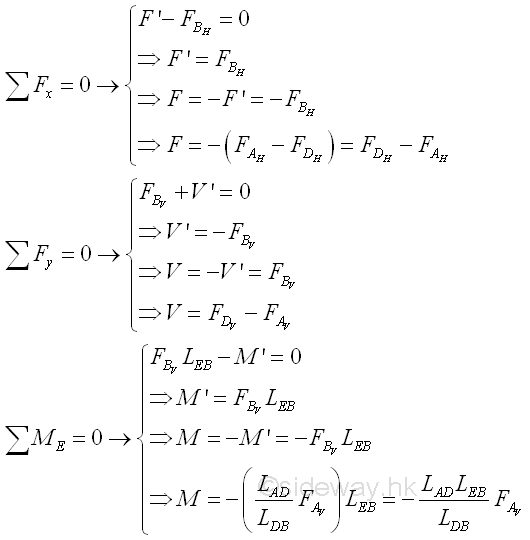Therefore the internal forces at point E is not the same the internal forces at point C .

### Two-force Frame Member

For a two-force frame member, although the external forces acting on the frame member should be equilibrium when the frame member is in equilibrium, the internal forces in the two-force frame member  also varies with the location of the point of interest if the two-force frame member is not a straight member. The internal force of the two-force frame member can also be determined by assuming the member is being cut by an imaginary section plane. For example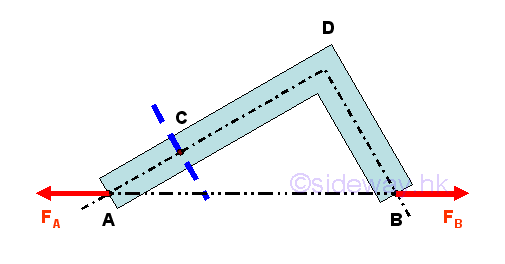Since the frame member is in equilibrium, tension force F at point A and tension force F' at point B should be equal in magnitude but opposite in sense. For a frame member in equilibrium, the internal forces at the imaginary section plane C should also be in equilibrium. Therefore, the frame member ADB can be considered as two separated equilibrium free body models, member section AC and member section CDB. Imply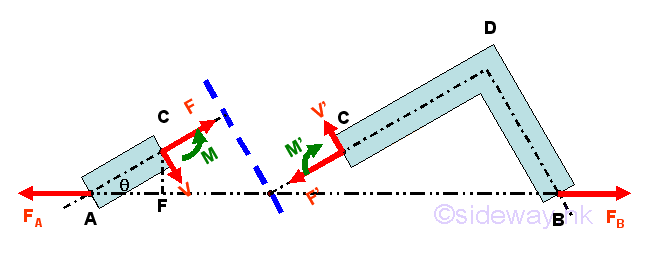Since the two seperated equilibrium free bodies, member section AC and member section CDB are in equilibrium, the internal forces reacts by the imaginary section plane C on member section AC and member section CDB must equal to external forces on each member. Therefore a system of forces which equivalent to the internal force and is equal and opposite to the system of forces due to force FA should be applied to member section AC at C. And a system of forces which equivalent to the internal force and is equal and opposite to the system of forces due to force FB should be applied to member section CB at C. The internal forces on the two seperated member sections AC and CDB should alway exert on each other in frame member AB and are equal in magnitude but opposite in sense. For the two-force frame member, the internal forces are a system of forces and moment which can be transformed to a force-couple system along the axis of the two-force frame member, the location of the imaginary section plane C will affect the magnitude of the force-couple system of the internal forces in member AB because the resultant moment of the internal forces is affected by the location of interest at which moment of the external forces acting on and the resultant force of the internal forces is also affected by the relative position of application of the external forces.

Consider the frame member section AC of length LAC, the internal forces at point C are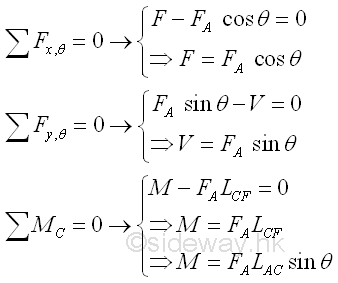Therefore the internal forces of the frame member section AC varies with the position of interest in the frame member AB.

©sidewayReferences

1. I.C. Jong; B.G. rogers, 1991, Engineering Mechanics: Statics and Dynamics, Saunders College Publishing, United States of America
2. F.P. Beer; E.R. Johnston,Jr.; E.R. Eisenberg, 2004, Vector Mechanics for Engineers: Statics, McGraw-Hill Companies, Inc., New YorkID: 120800020 Last Updated: 2012/8/22 Revision: 0 Ref:Home (5)

Business

Management

HBR (3)

Information

Recreation

Hobbies (7)

Culture

Chinese (1097)

English (336)

Reference (66)

Computer

Hardware (149)

Software

Application (187)

Digitization (24)

Numeric (19)

Programming

Web (644)CSS (SC)

ASP.NET (SC)

HTML

Knowledge Base

Common Color (SC)

Html 401 Special (SC)

OS (389)MS Windows

Windows10 (SC)

.NET Framework (SC)

DeskTop (7)

Knowledge

Mathematics

Formulas (8)

Number Theory (206)

Algebra (20)

Trigonometry (18)

Geometry (18)

Calculus (67)

Complex Analysis (21)

Engineering

Tables (8)

Mechanical

Mechanics (1)

Rigid Bodies

Statics (92)

Dynamics (37)

Fluid (5)

Control

Acoustics (19)

Biology (1)

Geography (1)

Latest Updated Links

Copyright © 2000-2019 Sideway . All rights reserved Disclaimers last modified on 10 Feb 2019